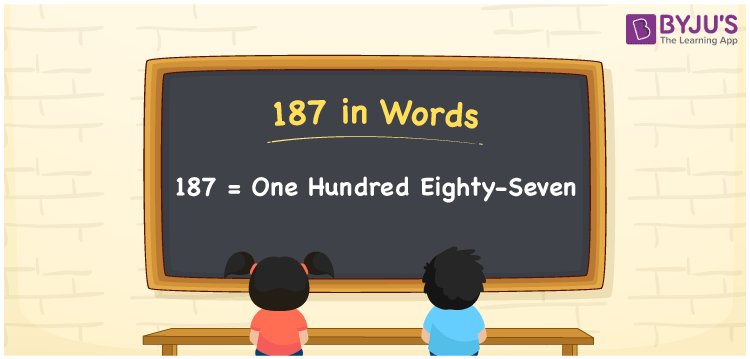# 187 in Words

187 in words is written as One Hundred Eighty-Seven. For example, Rohan gave Rs. 187 to his friend, then you can say, “Rohan gave Rupees One Hundred Eighty-Seven to his friend”. 187 is a cardinal number as it denotes a specific value of something. This article explains how to write the number 187 in words effortlessly using a place value method.

 187 in Words One Hundred Eighty-Seven One Hundred Eighty-Seven in numerical form 187

## 187 in English Words

English is the most widely used language in education. Thus, in Mathematics, we write numbers in words with the help of the English alphabet. Therefore, the number 187 in words is written as One Hundred Eighty-Seven.## How to Write 187 in Words?

187 can be written in words by knowing the position of each digit with the help of a place value system. We need to make a table of 3 columns since 187 is a three-digit number. The following table expresses the place value chart for the number 187.

 Hundreds Tens Ones 1 8 7

Hence, we can write the expanded form as:

1 x Hundred + 8 x Ten + 7 x One

= 1 x 100 + 8 x 10 + 7 x 1

= 100 + 80 + 7

= 187

= One Hundred Eighty-Seven

Therefore, 187 in words is written as One Hundred Eighty-Seven

Interesting way of writing 187 in words

1 = One

18 = Eighteen

187 = One Hundred Eighty-Seven

Thus, the word form of the number 187 is One Hundred Eighty-Seven

187 is a natural number that precedes 188 and succeeds 186

• 187 in words – One Hundred Eighty-Seven
• Is 187 an odd number? – Yes
• Is 187 an even number? – No
• Is 187 a perfect square number? – No
• Is 187 a perfect cube number? – No
• Is 187 a prime number? – No
• Is 187 a composite number? – Yes

## Frequently Asked Questions on 187 in Words

Q1

### How do you write 187 in words?

187 in words is written as One Hundred Eighty-Seven.
Q2

### Simplify 170 + 17, and express in words.

Simplifying 170 + 17, we get 187. Therefore, the number 187 in words is One Hundred Eighty-Seven.
Q3

### 187 is an odd number. True or False.

True, the number 187 is an odd number.